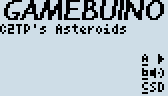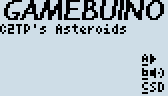# How to optimize game code properly?

So I’ve made my own Asteroids game and works pretty well, until after I’ve got more than 3 asteroids on the screen it becomes slower… Would there be anyone here able to check my code and see what I could do to speed things up a bit? Here’s what the game looks like so far anyway1 Like

@Craig_Page, congratulations, this game looks neat!How are you generating those sprites, is that some kind of vector graphics generation?

Thanks!
They’re drawn using the drawLine commands. Imagine stars * that have random length of lines which is not drawn, and then lines to join the end of each line to another.
These points are then manipulated by angle using cos and sin.
The ship uses the same method, except it’s given fixed points for drawing the lines instead of being random like the rocks.

1 Like

That’s quite cool, well done!

I’m really swamped with work right now and will try to review your code as soon as possible.
If somebody else on the forum is willing to take a look at @Craig_Page’s code, that would be much appreciated!

If you’re manipulating the points by cos and sin, then does that mean you’re working with floats? Using floating-point numbers can cause a big performance hit because the ATmega328 in the Makerbuino doesn’t have native floating-point support. It might be hard to switch to using fixed point if you still want to use sin and cos, though, as you might have to do some extra research to find a library or algorithm to get that working.

Hopefully, there’s a simpler optimization that could be made in your code, but there’s no way to know without being able to check it out. Do you not want to share it publicly yet?

1 Like

I can post the code in question:

``````class Shape
{
public:
Shape() {};
Shape(byte size) {
for (int i = 0; i < MAX_SHAPE_POINTS; i++) {
point[i] = size;
}
}
void setPointSize(int i, byte newSize) {
point[i] = newSize;
}

void draw(float x, float y) {

for (int i = 0; i < MAX_SHAPE_POINTS; i++)
{
word fixedpa = 360 / MAX_SHAPE_POINTS;

/*pa Point Angle, sa Shape Angle*/
word pa = angle + (fixedpa * i);
float sa = pa * M_PI / 180;
byte size = point[i];

int lx1 = x + (cos(sa)*size);
int ly1 = y + (sin(sa)*size);
//gb.display.drawLine(x, y, lx1, ly1);

if (i + 1 < MAX_SHAPE_POINTS) {
pa = angle + (fixedpa * (i + 1));
size = point[i + 1];
}
else {
pa = angle;
size = point;
}
sa = pa * M_PI / 180;

int lx2 = x + (cos(sa)*size);
int ly2 = y + (sin(sa)*size);
//gb.display.drawLine(x, y, lx2, ly2);

gb.display.drawLine(lx1, ly1, lx2, ly2);

}
}
void incAngle(float step) {
//if (angle + step <= 0) { angle += 360; }
angle += step;
//while (angle >= 360) { angle -= 360; }
}
float getAngle() {
return angle;
}
void setAngle(float newAngle) {
angle = newAngle;
}

private:
float angle = 0;
byte point[MAX_SHAPE_POINTS + 1];
float speed;
};
``````

I’ve noticed I have used floats a lot here… I’m thinking I may have to redo the entire thing using ints? Or I can see about improving performance by reducing the number of points drawn per shape…

I’ve looked into optimising methods on the wiki, but bit pushing/pulling may be a bit too complicated… (mind the unintended pun) However I now understand that multiplying and division doesn’t really help much in this situation. Maybe I’m making something that maybe a bit much for the MAKERBuino?

I’ve had a look through and made a few changes to my code, I’ve also noticed I’ve used cos and sin an aweful lot in my code, which was called very often, almost on each game loop. So I’ve made fixes where it would only rotate or change angles of the rocks every so often, not every loop.

I’ve also made some more progress to the game! New sparkly bits… I’ve also reduced the number of points for the rocks to draw… it helped improve performance somewhat2 Likes